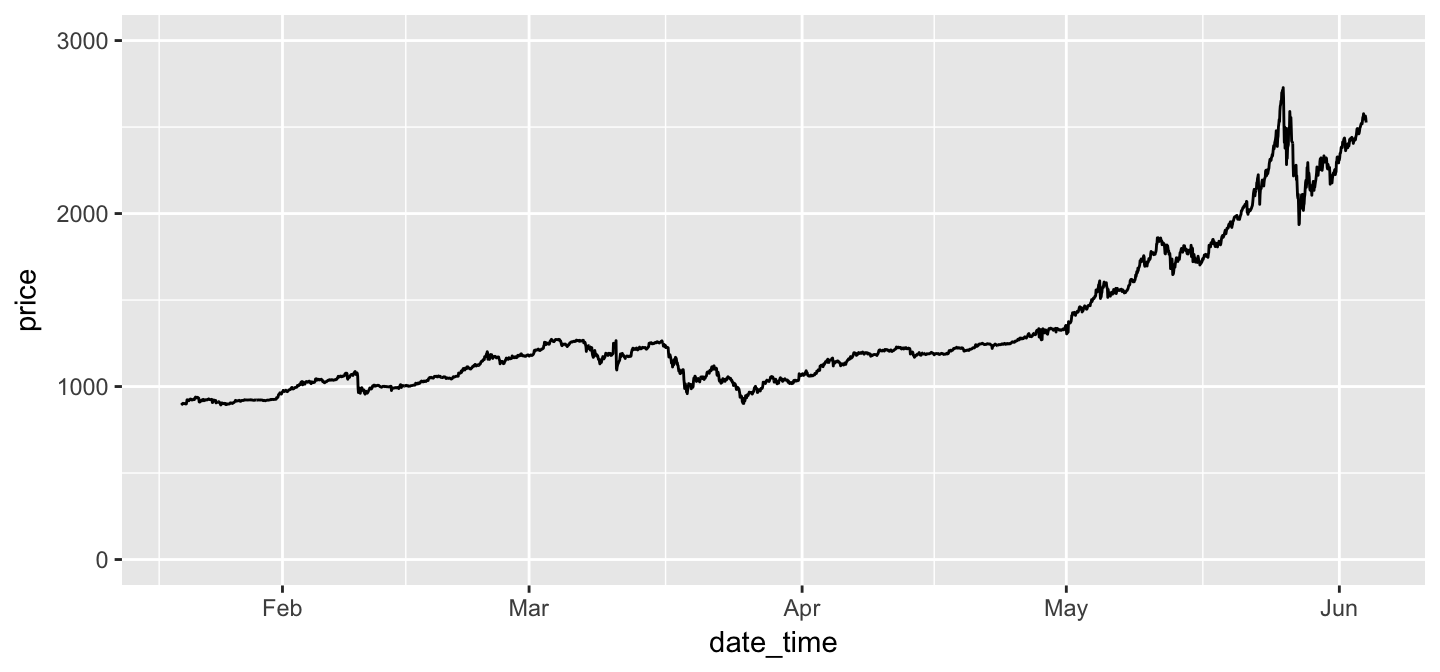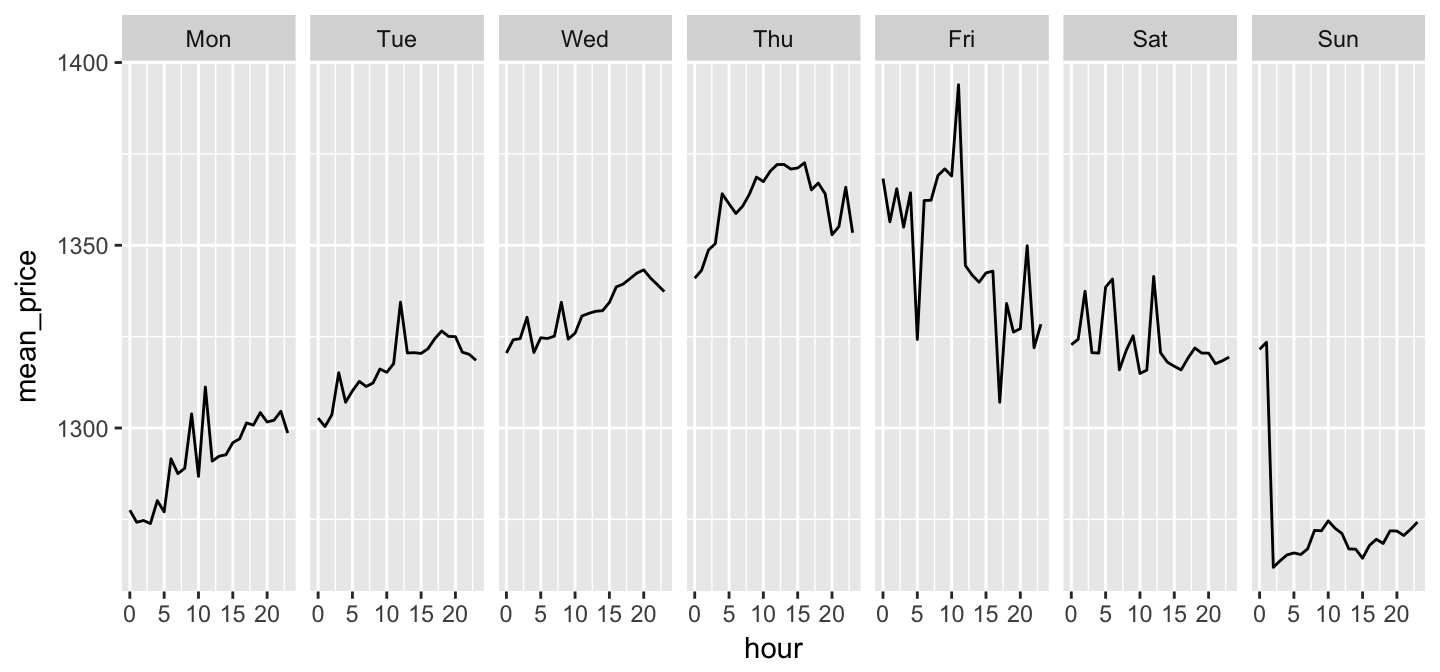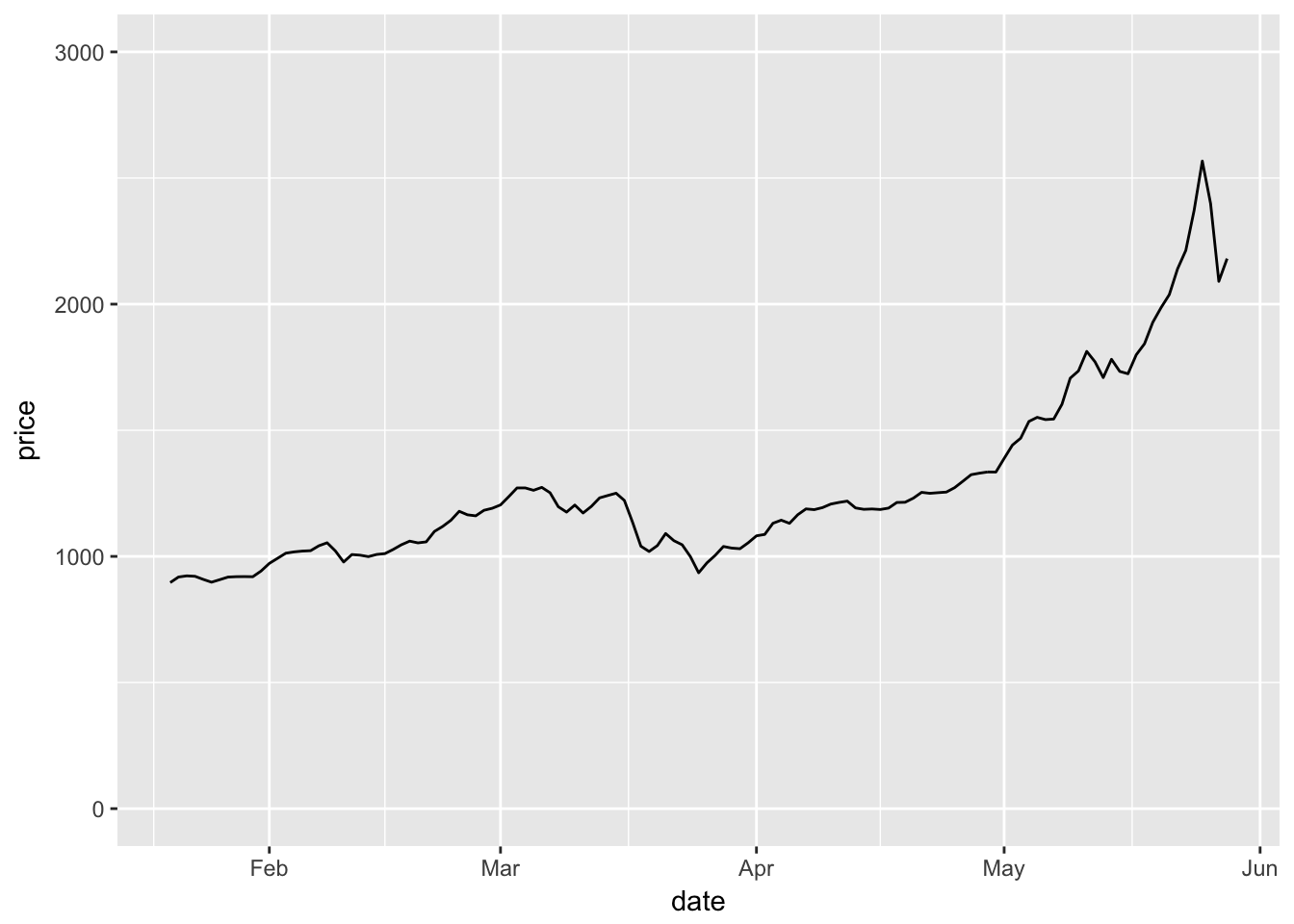dec/2017 update: @bitcoinprice stopped tweeting on June 4, 2017 --- the day after I posted this. Interesting...
Coincidence?! Probably.

• part1: quick description of idea, plot + validate twitter btc price strategy (just twitter + external btc data)
• part2: use monkeylearn classifiers to measure sentiment abt stuff, moving to btc topic (introduce monkeylearn api)
• part3: identify key dates, assess rel’ship btwn btc sentiment and price (limitations of twitter api become obvious here)

The use of twitter data for estimating public sentiment about issues can still be considered a fledgling-ish field. Here we assess the degree to which a fairly naive sentiment classifier can be used to predict the trajectory of the average bitcoin trading price, over time. The catch is that we’ll only use data publicly available via the twitter API. This is limiting in a few respects, chief among which is that targeting specific historical date ranges is basically impossible with only twitter's public search API tools. A scaled-up (and idealized) version of this strategy would use firehose output for bitcoin-related keywords at particular time intervals during which bitcoin trading prices are volatile.

A few links to stuff used or mentioned in this post:

### 1. btc prices from twitter

``````# load dependencies:

# - for data acquisition and processing
library("lubridate"); library("reshape2"); library("dplyr")

# - for displaying results
library("ggplot2"); library("scales"); library("knitr")

# set auth cache to false to avoid prompts in `setup_twitter_oauth()`
options(httr_oauth_cache=FALSE)``````
``````# read in api keys and access tokens
# set up twitter auth -- call `setup_twitter_oauth()` w keys from `keyz`
consumer_key    = keyz\$value[keyz\$auth_type=="twtr_api_key"],
consumer_secret = keyz\$value[keyz\$auth_type=="twtr_api_sec"],
access_token    = keyz\$value[keyz\$auth_type=="twtr_acc_tok"],
access_secret   = keyz\$value[keyz\$auth_type=="twtr_acc_sec"]
)``````
``##  "Using direct authentication"``

#### get btc price tweets + clean them up

The user @bitcoinprice is a bot that tweets out the average BTC trading price on an hourly basis. We want to build an hourly time-series dataset of BTC prices, starting right now and going back as far as the API will let us go.

``````# want days to be formatted as default abbrevs, but ordered like this
day_labels <- c("Mon","Tue","Wed","Thu","Fri","Sat","Sun")

# query the API for max allowed num of tweets (3200) from user @bitcoinprice,
# then clean up the data, toss all the info we don't want/need, + call it `dat`
dat <- userTimeline(user="bitcoinprice", n=3200, excludeReplies=TRUE)  %>%
# convert json-ish tweets to a df
twListToDF() %>%
# not interested in retweeted content
filter(!isRetweet) %>%
# make time-series easier to deal with
mutate(date = date(created),
hour = hour(created),
date_time = as.POSIXct(created, tz="UTC")) %>%
# get day of the week, give them quick labels as defined above
mutate(day = weekdays(date_time, abbreviate=TRUE)) %>%
mutate(day = factor(day, levels=day_labels)) %>%
# put colnames in my preferred format
rename(data_src = screenName,
num_fav = favoriteCount,
num_RT = retweetCount,
is_RT = isRetweet) %>%
# toss everything that's not relevant, arrange as desired
select(data_src, date_time, date, hour, day,
num_fav, num_RT, is_RT, text)``````

The tweets – now in `dat\$text` are completely formulaic, and have the following shape:

@bitcoinprice: The average price of Bitcoin across all exchanges is <dddd.dd> USD

where <dddd.dd> is the string that we want to extract and convert to numeric. We’ll extract it with some straightforward regex-ing, performing a couple of quality control checks along the way.

``````# a tweet consists of: toss + "dddd.dd" + toss
toss <- c("The average price of Bitcoin across all exchanges is ", " USD")

# check that all tweets are formulaic + as expected
if (!all(grepl(paste0(toss, "\\d*\\.?\\d*", toss), dat\$text))){
warning("careful -- tweets not as formulaic as they may seem! :/ ")
}

# given formulaic tweets, remove `toss` + extract price from `dat\$text`
dat\$price <- gsub(paste0(toss, collapse="|"), "", dat\$text, perl=TRUE)

# check we don't have any words/letters left before converting to numeric
if (sum(grepl("[a-z]", dat\$price)) > 0){
warning("careful -- some tweets were not in expected format! :o ")
}

# now convert to numeric
dat\$price <- as.numeric(dat\$price)``````

Quick inspection reveals that there’s something anomalous about one of the tweets: on 2017-03-14 15:00:03, @bitcoinprice says the average trading price is \$1.25. A cursory glance at the surrounding data points suggests that it’s probably meant to be \$1225.00.1

``````# fix the incorrect data point
dat\$price[dat\$price==1.25] <- dat\$price[dat\$price==1.25] * 1000``````

And make a quick table for a final quality check – seems reasonable for the last 4-ish months of BTC pricing.

``````kable(table(cut(dat\$price, breaks=seq(0, max(dat\$price), 500))),
col.names=c("price range (USD)","count"))``````
price range (USD) count
(0,500] 0
(500,1e+03] 478
(1e+03,1.5e+03] 1977
(1.5e+03,2e+03] 407
(2e+03,2.5e+03] 301

#### plot the data

Now we can look at the price of BTC over recent months, using @bitcoinprice’s tweets. We’ll validate the data with an external source in the next step.

``````# what's our date range? (3200 hourly tweets so should be 3200/24 days ~ 4mo)
range(dat\$date)``````
``##  "2017-01-20" "2017-06-04"``
``````# make some plots

# price over time for full range of tweets
ggplot(dat, aes(x=date_time, y=price)) +
geom_line() + scale_y_continuous(limits=c(0, 3000))````````````# aggregate over day-hour pairs to look at typical structure of a week
pdat <- dat %>% select(day, hour, price) %>% group_by(day, hour) %>% summarize(
mean_price = mean(price)
) %>% data.frame()

ggplot(pdat, aes(x=hour, y=mean_price)) +
geom_line() +
facet_wrap(~day, ncol=7)``````#### inspect + validate accuracy on external data_src

Just to make sure our prices are accurate, we can validate them on an external source of data. Here we’ll use bitcoinity.org, which offers a nice little data export feature on the price-tracking portion of their website.

``````# get external price data from bitcoinity.org
"data/bitcoinity_data-price-volume.csv",
col.names=c("date","price","volume"),
colClasses=c("Date","numeric","numeric")
)
bitcoinity\$data_src <- "bitcoinity.org"

date price volume data_src
2010-07-17 0.0495100 0.990200 bitcoinity.org
2010-07-18 0.0779750 5.091994 bitcoinity.org
2010-07-19 0.0867925 49.731775 bitcoinity.org
2010-07-20 0.0779994 20.595480 bitcoinity.org

Now plot the bitcoinity data on the same interval as the @bitcoinprice data. The result is sparser than the above plot since there’s fewer data points per 24-hr period, but the pattern looks identical. Nice.

``````# the external data, plotted on the same interval as `dat` above:
ggplot(bitcoinity[bitcoinity\$date >= min(dat\$date), ], aes(x=date, y=price)) +
geom_line() + scale_y_continuous(limits=c(0, 3000))``````Just for reference, here’s the trajectory of BTC since the very beginning (fml can’t believe I didn’t get in when I first heard about BTC back in 2011 :/). There’s also a trading volume column in the bitcoinity data, which we’ll plot in orange in the same window.

``````# want to display the all-time high price and date
fig_cap <- paste0(
"all-time high: \$", round(max(bitcoinity\$price)), ", on ",
bitcoinity\$date[bitcoinity\$price==max(bitcoinity\$price)]
)

# the external data, plotted on the whole lifetime of btc:
ggplot(bitcoinity, aes(x=date, y=price)) +
geom_line() +
geom_line(aes(x=date, y=volume/1e6), color="#ed9953") +
geom_hline(yintercept=max(bitcoinity\$price),
color="#8aa8b5", linetype="dashed") +
annotate(geom="text", x=as.Date("2016-02-01"), y=max(bitcoinity\$price)+100,
color="#8aa8b5", label=fig_cap) +
scale_y_continuous(limits=c(0, 3000)) +
scale_x_date(date_breaks="6 months") +
theme(axis.text.x=element_text(angle=45, vjust=1, hjust=1),
plot.caption=element_text(color="#ed9953", hjust=0)) +